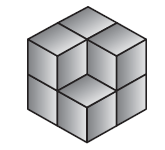# Stage 3 Year 5 Measurement

43 Questions | Total Attempts: 347SettingsYear 5 Measurement

• 1.
12345mL=____________L    Only write the number.   No spaces between the numbers.
• 2.
276mL = ________________L
• 3.
109mL = __________L
• 4.
826L = ____________mL
• 5.
12L = ____________mL
• 6.
3.89L = ____________mL
• 7.
Label this cubic centimetre model with its volume, capacity and appropriate unit.
• A.

Volume 7cm cubed, 70mL, 70g

• B.

Volume 7cm cubed, 700mL, 700g

• C.

Volume 7cm cubed, 7mL, 7g

• 8.
What would happen to the level of water in the jug if this centicube model is placed inside:
• A.

The level of the water would stay the same.

• B.

The level of the water would rise from 400 mL to 440 mL

• C.

The level of the water would rise from 400 mL to 404 mL

• D.

The level of the water would rise from 400 mL to 444 mL

• 9.
Calculate 10 x 75 kg = ______kg Only write the number.   No spaces between the numbers.
• 10.
600 kg + _________kg  = 1t
• 11.
A 50 seater aeroplane was filled to capacity. The passengers had an average mass of 74 kg. What is the total mass of the passengers in tonnes?___________tonnes
• 12.
_________cm Only write the number.   No spaces between the numbers.
• 13.
Jenna runs at a speed of 6 km per hour. How long would it take her to run 3 km?
• A.

30 minutes

• B.

40 minutes

• C.

50 minutes

• D.

60 minutes

• 14.
Which is the coldest temperature?
• A.

7 degrees celcius

• B.

18 degrees celcius

• C.

-4 degrees celcius

• D.

-14 degrees celcius

• 15.
You need to catch a bus at 11.50 am. You know you have 20 minutes before you need to leave. What time is it?
• A.

A

• B.

B

• C.

C

• D.

D

• 16.
What was the average time for the training sessions?
• A.

50 minutes

• B.

30 minutes

• C.

20 minutes

• D.

40 minutes

• 17.
• A.

8 cm squared

• B.

12 cm squared

• C.

14 cm squared

• D.

16 cm squared

• 18.
Only write the number.   No spaces between the numbers.
• 19.
Tim started his homework at 4:23 pm and finished it at 6:20 pm. How long did he spend doing his homework?
• A.

2 hours and 3 minutes

• B.

1 hour and 3 minutes

• C.

1 hour and 57 minutes

• D.

2 hours and 43 minutes

• 20.
• A.

4.8 metres

• B.

4 metres

• C.

5 metres

• D.

4.5 metres

• 21.
A length of ribbon 3.6 metres long is cut into pieces that are 0.06 long. How many pieces of ribbon can be cut?
• 22.
Compare Paris and New York
• A.

Sunday

• B.

Monday

• C.

Thursday

• D.

Friday

• 23.
• A.

1.2cm

• B.

1.6cm

• C.

2.6cm

• D.

0.6cm

• 24.
Tina bought 35 L of petrol at \$1.52 per litre. How much did she pay for the petrol? Put the dollar and decimal point sign no gaps.
• 25.
Related TopicsBack to top